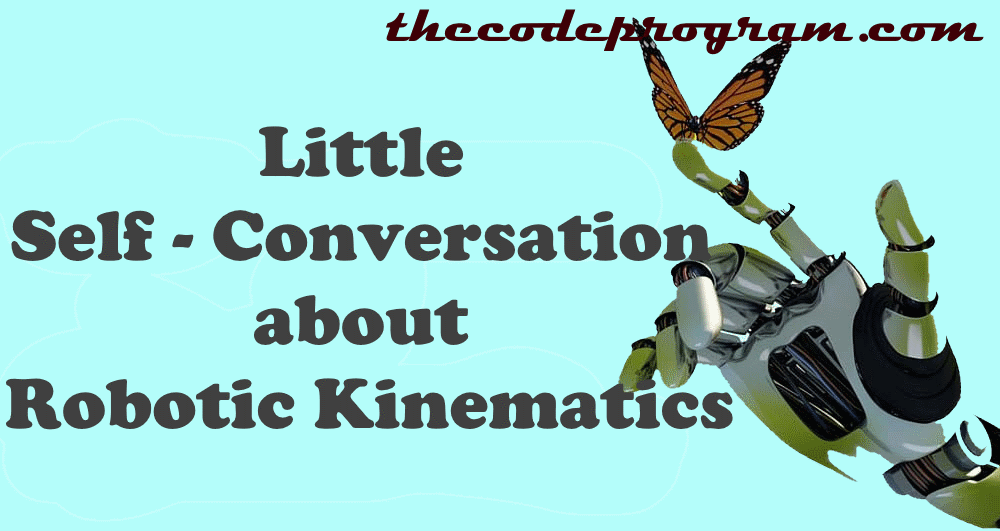## A Little Self-Conversation about Robotic Kinematics

Hello everyone, in this article we are going to talk about Forward Kinematics. We will answer the What is Forward kinematics, what its purpose of it and where can be used questions.

Let's begin.

Firstly I want to talk about what is kinematic?

Kinematic is a subfield of Mechanical in Physics. It exemines the motion and acceleration and do not care about mass ad force. When the movement started the kinematics being started. We can describe the movement as a body or a point going another location that differant from initial position. In kinematics the Geometric arguments like initial position and the target location is required for robotic operations.

So, Let's start to talk about Forward Kinematics:

Forward kinematics refers to use the kinematic equasitions with geometric values of the support parts ant its joints to calculate the final position of a system. Forward kinematics using in robotics, animations and game development areas occasionally. The reverse calculation of Forward Kinematic is called as Inverse Kinematics. With Inverse kinematics our parameters will be target position and we calculate the geometric values of supports and joints.

If you are working robotic systems or 3D motion systems, you will have conversation with your teammate or yourself, I / we have to calculate the Kinematics of this system. At this point first you will have to decide how many joints do you have ?

If you have only two connection points with variable lenght linear actuators, so many system using the Inverse Kinematics to reach target position. But if you have more connection points you need to use Forward Kinematics.

6DOF stewart platforms can be developed with linear actuators and fixed rotary actuators. I researched so many sources and as I see if there are liear actoators used, Inverse kinematics is the easier than forward kinematics. But if have more joints Inverse kinematics getting hard so much. But it does not mean you can not use it. You can use which method is appropraite for you.

Below image you will see the differances between Stewart Platforms with Two joints and more than two joints.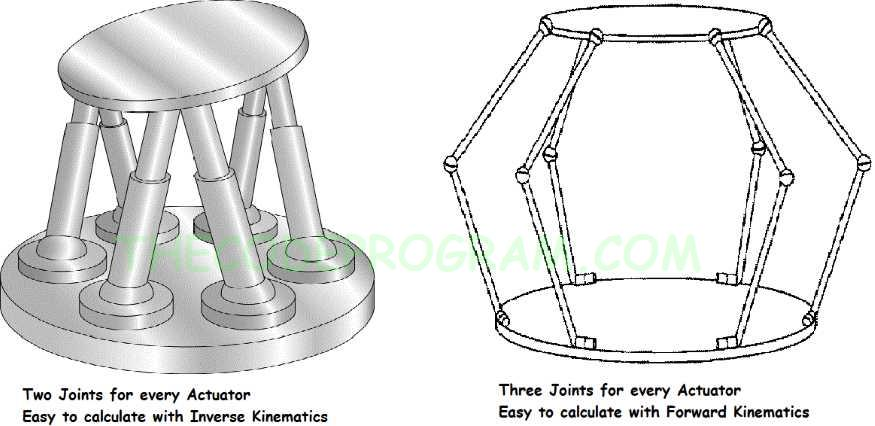#### So, How can we make a kinematic calculation?

I assume you have what Tait-Bryan and Euler Transformational Matrixes are. Also you can solve some trigonometric equasitions.

Also Taking notes and specifing the basics of the movement platform first will be good for you. Because calculating the kinematics is not simple, this means you may experience some confuses during the building the movement system.

Let's First take a look at Forward Kinematics.

If we are ready, we must first specify the axis and geometric values of our platform. These values will be our initial values for calculating. And then we need to specify the desired geometrical position.

I am using the Right Hand rule to specfy the Cartesian Axes. According to right hand rule the fingers will show the axis like below image.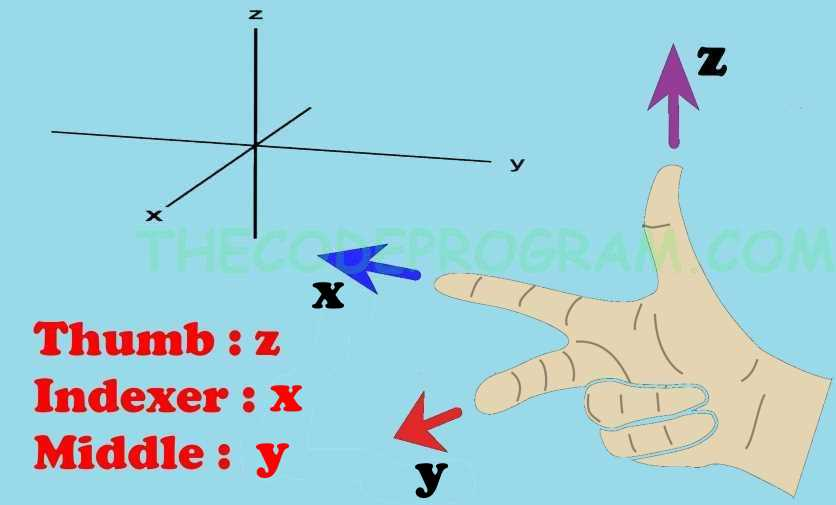• Thumb Finger z-axis
• Middle finger y-axis
• Indexer finger x-axis

After these we need to specify our End Effector position. We need the know before parts geometrical positions to calculate End Effector position. All of them parts have lenght values and relative angle values to the before part. During calculatin of the end effector position we use the matrixes to make the process tidied up.

Below image you can see the simple schematic of a robotic arm.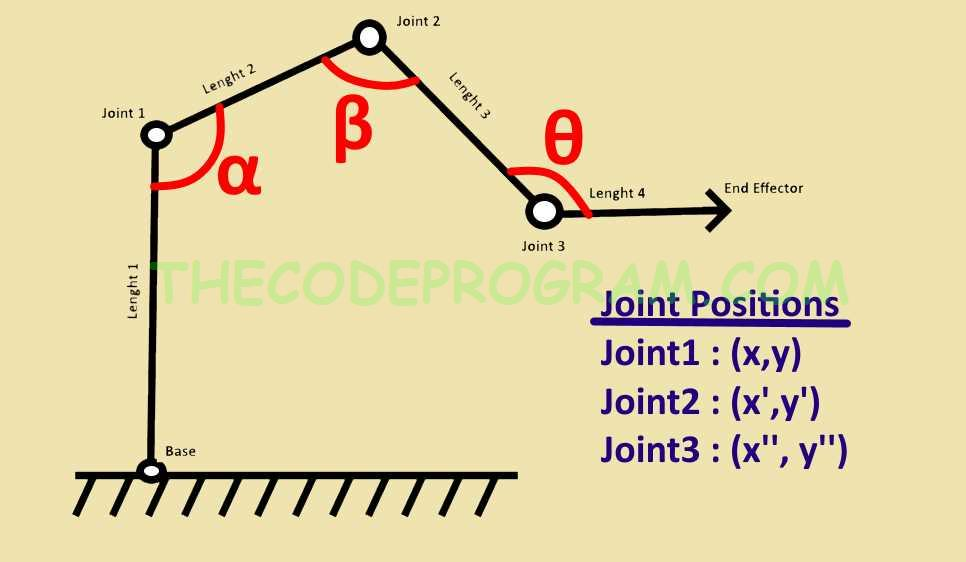In the above image there are more than two joints. So applying the Forward kinematic solution will make our task easy.

To apply forward kinematic we need to use matrixes in order from the where the movement begin to the end effector.

Normal method of the equation is using rigid transformation [Z] to characterize the relative movement allowed at each joint and separate rigid transformation [X] to define the dimensions of each link. The result is where [T] is the transformation locating the end-link.
``````
[T]=[Z1]*[X1]*[Z2]*[X2]*.....*[X(n-1)]*[Z(n)
``````

In 1955 Denavit and Hartenberg standardized joint matrices [Z] and link matrices [X] with frame matrixes. We also have in this standart Z-axis movement.

According to this related standardisation :

``````
[Z(i)]=Trans(Z(i)(d(i)) * Rot(Z(i)(theta(i)),
``````

Generally Denavit - Hartenberg theory is using at robotic fields. To use this method we need to have some values in the system. These are :

For each joint :

• The angle value between current x-axis and previous x-axis according to z-axis
• Common normal states geometrical values.
• The distance between current x-axis and previous x axis around z-axis.
• The angle between current zaxis and previous z-axis around common normal line.
According to Wikipedia Common normal is : In robotics the common normal of two non-intersecting joint axes is a line perpendicular to both axes

What I understand from this phrase.

Common Normal is a distance between base and platform structures when they are paralel each other. Because when they parallel each other, they will never intersect.

End of the article we are going to see the basic matrix of the forward kinematics calculations. If you remember I talked a little bit about Tait-Bryan and Euler equations. Now it is time to use them. When we are dealing with 6DOF movement we have three rotational and three transitional axis. Take a look at these matrixes first.

Below you will see the rotational matrix of Tait-Bryan. This matrix refers to movement of a point at around all three axis.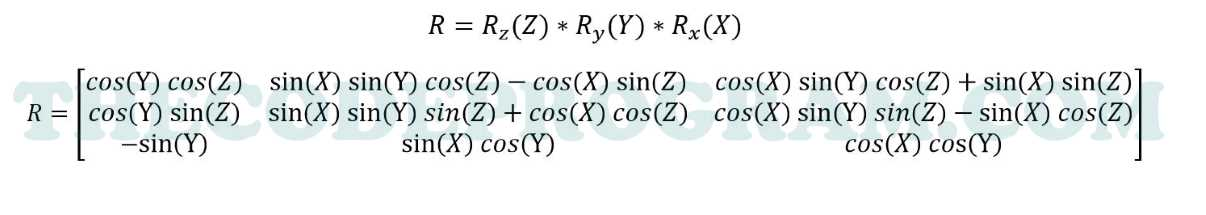But if you want to use Denavit - Hartenberg Forward Kinematics Solution, your End Effector matrix will be like below.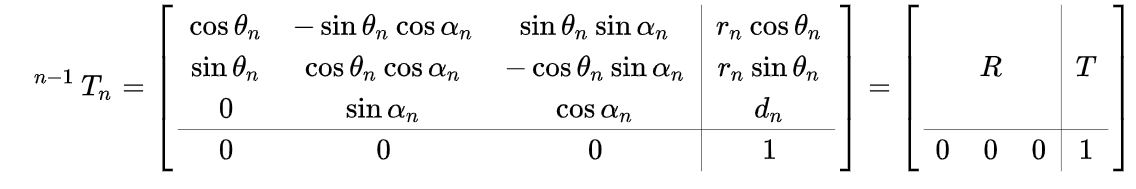As you can see there are multiple ways to make calculations.

Conclusion

With forward and inverse kinematics you can build a 6dof movement solution. Look around and you will it these calculations at everywhere. When you play a 3D mobile racing game or when you see a quadcopter is flying. All of movement parameters are calculating with kinematics. According to these parameters, servos or brushless motors are revoluting and somethings changing.

You can see kinematics everywhere.

Have a good Kinematics Calculations.

Burak Hamdi TUFAN.

03/04/2020

02/04/2020

20/10/2021